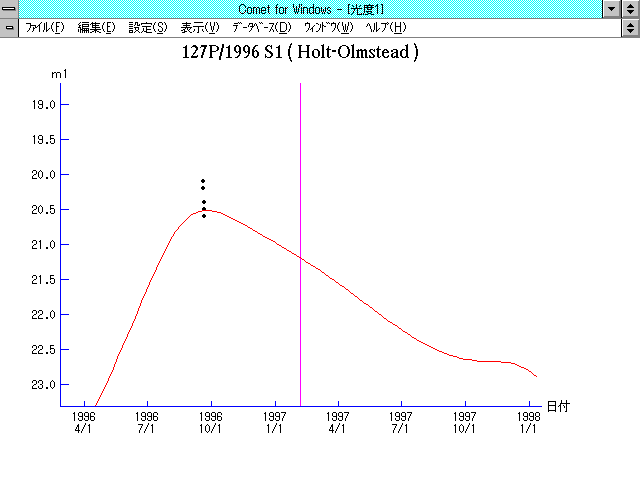# \$B%[%k%H(B-\$B%*%k%`%9%F%C%IWB@1(B###\$B%W%m%U%#!<%k(B

 \$BId9f(B 127P/1996 S1 \$B8!=PF|(B 1996\$BG/(B9\$B7n(B19\$BF|(B \$B8!=P8wEY(B 20.2\$BEy(B \$B8!=P J. V. Scotti (Kitt Peak)

###\$B50F;MWAG(B

```   The following improved orbital elements, by Kenji Muraoka, are from
44 observations 1990 Sept. 16 to 1996 Sept. 20, perturbations by 9
Planets, Moon and 5 minor planets were taken into account.
The mean residual is +/- 0.70 arc seconds.

Epoch  =  1997 Feb.  1.0  TT       JDT = 2450480.5
T  =  1997 Feb.  6.66053       +/- 0.00375 (m.e.) TT
Peri. =    6.25686                +/- 0.00145
Node  =   14.06115                +/- 0.00009   (2000.0)
Incl. =   14.40683                +/- 0.00011
q  =    2.1534991              +/- 0.0000078 AU
e  =    0.3704719              +/- 0.0000024
a  =    3.4208151              +/- 0.0000052 AU
n  =    0.15577927             +/- 0.00000036
P  =    6.327                  +/- 0.0000146  years
```

###\$B@1?^(B1996\$BG/(B 7\$B7n(B 6\$BF|!A(B1997\$BG/(B12\$B7n(B28\$BF|(B

###\$B8wEYJQ2=(B

```        m1 = 14.0 + 5 log\$B&\$(B + 15.0 log r
```##### \$B50F;MWAG\$OB<2,7r<#;a\$N7W;;\$K\$h\$k\$b\$N\$G\$9!#(B \$B@1?^\$O(B StellaNavigator Ver.2.0 for Windows (\$B%"%9%H%m%"!<%D(B \$BJTCx(B / \$B%"%9%-!<=PHG6I4)(B) \$B\$G:n@.\$7\$?\$b\$N\$G\$9!#(B \$B8wEY%0%i%U\$O(BComet for Windows\$B\$G:n@.\$7\$?\$b\$N\$G\$9!#(B